Thermodynamics and Propulsion

# 6.3 Representation of Thermodynamic Processes incoordinates

It is often useful to plot the thermodynamic state transitions and the cycles in terms of temperature (or enthalpy) and entropy,,, rather than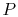,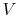. The maximum temperature is often the constraint on the process and the enthalpy changes show the work done or heat received directly, so that plotting in terms of these variables provides insight into the process. A Carnot cycle is shown below in these coordinates, in which it is a rectangle, with two horizontal, constant temperature legs. The other two legs are reversible and adiabatic, hence isentropic (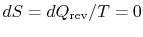), and therefore vertical in-coordinates.If the cycle is traversed clockwise, the heat added is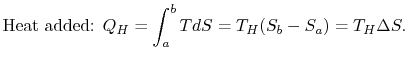The heat rejected (from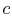to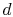) has magnitude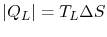.

The work done by the cycle can be found using the first law for a reversible process: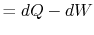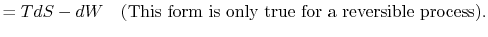We can integrate this last expression around the closed path traced out by the cycle:Howeveris an exact differential and its integral around a closed contour is zero: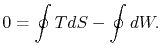The work done by the cycle, which is represented by the term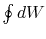, is equal to, the area enclosed by the closed contour in the-plane. This area represents the difference between the heat absorbed (at the high temperature) and the heat rejected (at the low temperature). Finding the work done through evaluation ofis an alternative to computation of the work in a reversible cycle from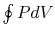. Finally, although we have carried out the discussion in terms of the entropy,, all of the arguments carry over to the specific entropy,; the work of the reversible cycle per unit mass is given by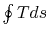.

Muddy Points

How does one interpret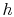-diagrams? (MP 6.3)

Is it always OK to switch''-and-diagram? (MP 6.4)

What is the best way to become comfortable with-diagrams? (MP 6.5)

What is a reversible adiabat physically? (MP 6.6)

UnifiedTP